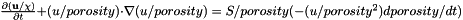Mushy Layer  1.0
mushyLayerOpt.h File Reference

Go to the source code of this file.

## Classes

struct  MushyLayerOptions
Contains most of the options for running the code, and how to handle the equations. More...

## Enumerations

enum  RefinementMethod {
tagSpeed, tagChannels, tagPlumeMush, tagMushChannels,
tagScalar, tagVector, tagMushChannelsCompositeCriteria
}
Different ways to add refinement. More...

enum  MGmethod { MGTypeStandard, MGTypeFAS }
Different multigrid methods. More...

enum  TaggingMethod { UndividedGradient, Magnitude, CompareLargerThan, CompareLargerThanNegative }
Strategy for tagging cells. More...

enum  RefluxMethod { LinearReflux, LinearVCReflux, NonlinearReflux }
Methods for doing implicit refluxing - i.e. what sort of equation to we solve for the correction. More...

Options for handling advection velocities, where S is the source term. More...

enum  PorosityFunctions {
constantLiquid, linear, gaussian, gaussianSinusoidal,
constantSmall, cubic, hyperbolicTan
}
Different options for enforcing a fixed porosity. More...

enum  VectorVars {
m_fluidVelAnalytic, m_fluidVelErr, m_dUdt, m_FsDiffusion,
m_FsFluid, m_Fs, m_freestreamCorrection, m_UpreProjection,
}
Cell centred vector field. More...

enum  ScalarVars {
m_enthalpy, m_bulkConcentration, m_temperature, m_porosity,
m_liquidConcentration, m_solidConcentration, m_pressure, m_permeability,
m_viscosity, m_lambda, m_lambda_porosity, m_enthalpySolidus,
m_enthalpyLiquidus, m_enthalpyEutectic, m_porosityAnalytic, m_temperatureAnalytic,
m_dHdt, m_dSdt, m_averageVerticalFlux, m_soluteFluxAnalytic,
m_verticalFlux, m_divU, m_averageHeatFlux, m_streamfunction,
m_vorticity, m_FsVertDiffusion, m_FsVertFluid, m_FsVertFrame,
m_divUcorr, m_pi, m_phi, m_MACBC,
m_MACrhs, m_CCrhs, m_passiveScalar, m_activeScalar,
m_lightIntensity, m_numScalarVars
}
Identifiers for different scalar variables. More...

## Detailed Description

Contains immunity flag definitions

## ◆ MGmethod

 enum MGmethod

Different multigrid methods.

Enumerator
MGTypeStandard

Normal linear multigrid.

MGTypeFAS

Full Approximation Scheme (for nonlinear problems)

## ◆ PorosityFunctions

 enum PorosityFunctions

Different options for enforcing a fixed porosity.

Enumerator
constantLiquid

Porosity = 1.0 everywhere.

linear

Porosity varies linearly with x,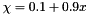.

gaussian

Porosity looks like a gaussian,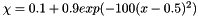.

gaussianSinusoidal

Gaussian with some sinuosoidal variation,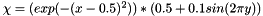.

constantSmall

Porosity = 0.1.

cubic

Porosity varies with the y-direction cubed,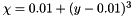.

hyperbolicTan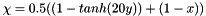## ◆ RefinementMethod

 enum RefinementMethod

Enumerator
tagSpeed

Tag where fluid speed exceeds threshold.

tagChannels

Tag where channels are.

tagPlumeMush

Tag where these is strong downflow in the mushy layer.

tagMushChannels

Tag porosity on level 0, and strong solute rich downflow thereafter.

tagScalar

Tag on some scalar field given by taggingVar.

tagVector

Tag on some vector field given by taggingVectorVar.

tagMushChannelsCompositeCriteria

Composite criteria for tagging cells.

Tag where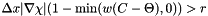where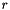is the refinement threshold,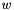is the vertical velocity,

## ◆ RefluxMethod

 enum RefluxMethod

Methods for doing implicit refluxing - i.e. what sort of equation to we solve for the correction.

Enumerator
LinearReflux

linear correction

LinearVCReflux

linear variable-coefficient correction

NonlinearReflux

nonlinear correction

## ◆ ScalarVars

 enum ScalarVars

Identifiers for different scalar variables.

Enumerator
m_enthalpy

Enthalpy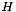.

m_bulkConcentration

Bulk concentration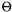.

m_temperature

Temperature,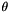.

m_porosity

Porosity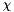.

m_liquidConcentration

Liquid concentration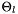.

m_solidConcentration

Solid concentration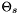.

m_pressure

Pressure used in the face centred projection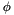.

m_permeability

Permeability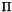.

m_viscosity

Viscosity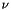.

m_lambda

Auxillary field lambda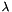for computing the freestream correction.

m_lambda_porosity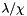m_enthalpySolidus

Solidus phase boundary,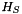.

m_enthalpyLiquidus

Liquidus phase boundary,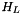.

m_enthalpyEutectic

Eutectic phase boundary,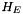.

m_porosityAnalytic

Some analytically calculated porosity field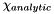.

m_temperatureAnalytic

Some analytically calculated temperature field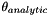.

m_saltEqnSrcGodunov

Explicit source term for the bulk concentration update.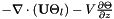m_Terr

Computes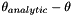if required.

m_enthalpySrc

Explicit source term for the enthalpy update.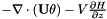Level divergence of the advection velocities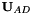.

m_dHdt

Rate of change of enthalpy with time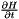.

m_dSdt

Rate of change of bulk concentration with time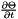.

m_averageVerticalFlux

Horizontally averaged vertical solute flux.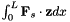m_soluteFluxAnalytic

Analytic solute flux for test problems.

m_verticalFlux

Vertical component of the solute flux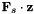.

m_divU

Level divergence of the cell centred velocity field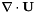.

m_averageHeatFlux

Horizontally averaged vertical heat flux.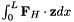m_streamfunction

Streamfunction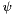(if calculated ad hoc during post processing)

m_vorticity

Vorticity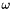(if calculated ad hoc during post processing)

m_pi

Pressure calculated in face-centred projection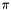.

m_phi

Pressure calculated in cell-centred projection.

m_MACBC

Coarse-fine boundary condition used in the face-centred projection.

m_MACrhs

Right hand side for the face-centred projection solve,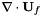.

m_CCrhs

Right hand side for the face-centred projection solve,.

m_passiveScalar

m_activeScalar

Active scalar which is advected and also has sources/sinks.

m_lightIntensity

Intensity of radiation from the surface.

m_numScalarVars

Number of scalars variables.

## ◆ TaggingMethod

 enum TaggingMethod

Strategy for tagging cells.

Enumerator

Tag where the undivided gradient of some field is bigger than some value.

Magnitude

Tag where the magnitude of some field is bigger than some values.

CompareLargerThan

Tag where the value of some field is larger than some criteria.

CompareLargerThanNegative

Tag where the value of some field multiplied by -1 is larger than some criteria.

Alternatively, can think of this as when some field is smaller than some criteria.

## ◆ VectorVars

 enum VectorVars

Cell centred vector field.

Enumerator
m_fluidVel

Cell centred fluid velocity,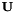.

m_U_porosity

Fluid velocity divided by porosity,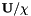.

m_Ustar

Unprojected cell centred fluid velocity,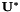.

Unprojected face centred fluid velocity, as used for advection, which has been averaged to cell centres.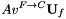Face centred fluid velocity, as used for advection, which has been averaged to cell centres.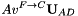m_UdelU

Inertial term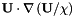.

Source term for the velocity advection.

m_numVectorVars

Number of vector variables.

Options for handling advection velocities, where S is the source term.

Enumerator
solve